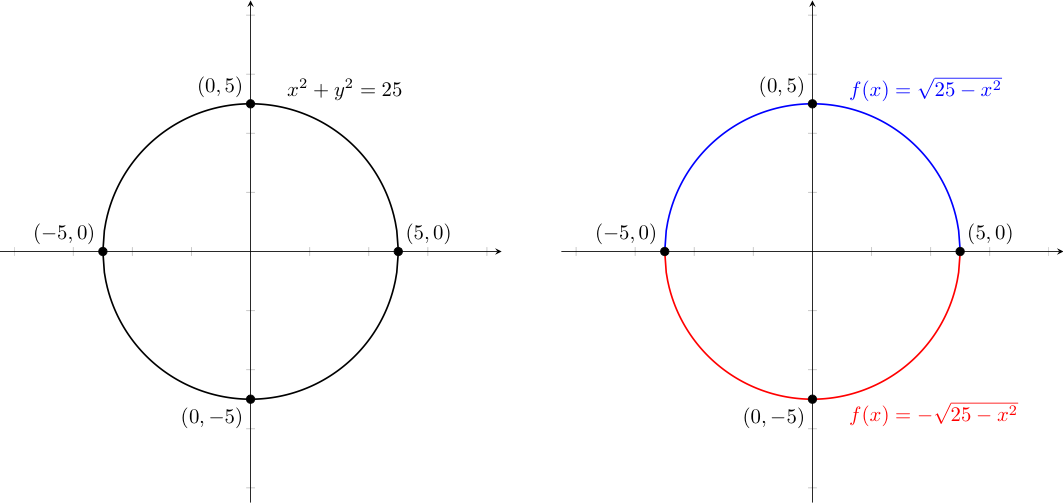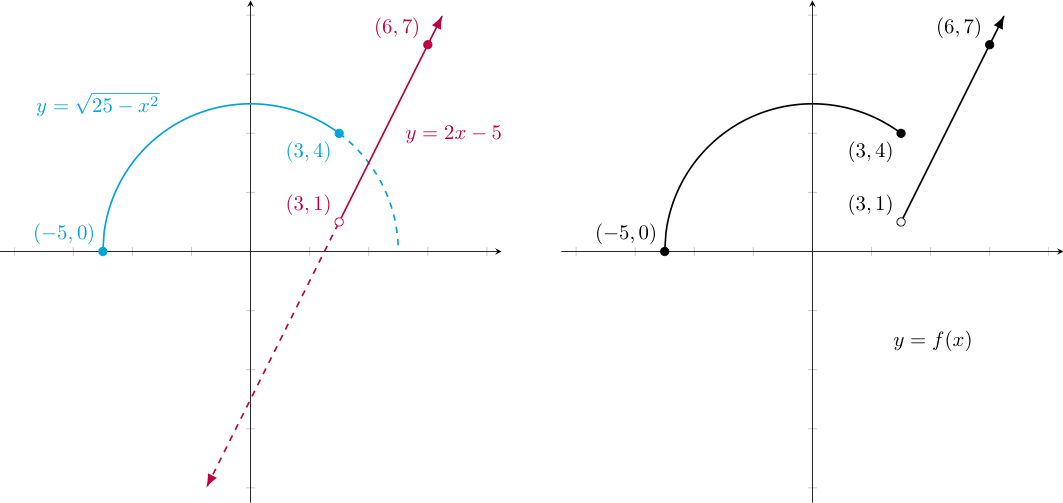## Restricting Relations and Piecewise-Defined Functions

As we have already seen, one can build new functions from others by combining them through either arithmetic operations (e.g., addition, subtractions, multiplication, and division) and through composition. In what's below, we explore two additional ways to build new functions.

### Restricting Relations

We have already seen how one can solve many equations that only involve a single variable for the value(s) of that variable that make the equation true. A natural question to then ask is what happens when an equation involves more than one variable?

For example, consider the simple equation $x^2 + y^2 = 25$. If we attempt to solve this equation for one variable, notice that for all $x \in (-5,5)$ there are two solutions for $y$. Likewise, for all $y \in (-5,5)$ there are two solutions for $x$. We can clearly say that neither $x$ or $y$ is a (single) function of the other. $$y = \pm \sqrt{25-x^2} \quad \textrm{ and } \quad x = \pm \sqrt{25-x^2}$$ That said, the $x$ and $y$ values that solve the equation discussed above are certainly related in some way. As such, we change our verbiage a bit. For any equation of two or more variables, the solution to that equation (i.e., the pairs of values that satisfy it) is called a relation.

One should note that just as we don't require an equation to describe a function (recall the peculiar way we described functions with arrows from one finite set to another, when we first introduced the notion of what a function was), we don't require an equation to describe a relation either. All we really need is a set of coordinate pairs (or $n$-tuples, more generally). Indeed, this is how a binary relation is typically defined: a set of ordered pairs, where we say $x$ and $y$ are related only if $(x,y)$ is in the aforementioned set.

† : In case you are curious, a set of $n$-tuples -- that is, coordinates in $\mathbb{R}^n$ (possibly representing solutions to an equation with $n$ variables, but not required to do so) can be used to define a more general $n$-ary relation.

Of course, defining such relations through the identification of a set of ordered pairs naturally leads to graphs of relations -- i.e., plots of the coordinate pairs that make up the relation on a coordinate plane.

In the case above, note that $x^2 + y^2 = 25$ precisely when $\sqrt{x^2 + y^2} = 5$. By the Pythagorean Theorem the left side of that second equation represents the distance between a point $(x,y)$ and the origin $(0,0)$. Thus, we see the graph of the relation $\{(x,y) \in \mathbb{R}^2 \ | \ x^2 + y^2 = 25\}$ is a circle of radius $5$, centered at the origin.

As an important aside, note that more generally -- but for similar reasons -- we can say: $$\textrm{The graph of }\{(x,y) \in \mathbb{R}^2 \ | \ (x-h)^2 + (y-k)^2 = r^2\} \textrm{ is a circle with center (h,k) and radius r}$$

Graphing $x^2 + y^2 = 25$ on the left below, note that it does not pass the vertical line test, which is consistent with our earlier observation that there is more than one $y$-value associated with several individual $x$-values (and vice-versa). As such, this relation can't be described by a single function.However, we can express this relation as the union of two function "pieces" -- shown here by considering those points with negative vs. non-negative $y$-values in the red and blue semi-circles above, respectively. Nicely, we can consider the points $(-5,0)$ and $(5,0)$ to be part of one or both pieces without affecting each piece's ability to be described as a function.

Importantly, while in this case it is easy to describe the blue and red functions using the formulas found when solving for $y$, it should be noted that not all equations in two variables $x$ and $y$ can be easily solved for $y$. So restricting relations really does expand the types of functions we can consider.

### Piecewise Functions

There are other ways to combine functions to create new ones. Recall when we earlier introduced the absolute value function, we made mention that it was "piecewise defined". Here we explore function of this type a bit further.

To create a "piece-wise defined" function, one breaks apart some domain into two or more disjoint pieces, using different functions to calculate the output for each $x$-value, where the function used is based upon the piece into which that particular $x$-value falls.

Again considering the absolute value function, $|x|$, recall that when $x \ge 0$, the absolute value function doesn't really do anything -- it returns its input unchanged. So, for this "piece" $|x| = x$.

However, when $x \lt 0$, the absolute value changes the sign of its input. Multiplying a value by negative one has the same effect -- so we can say that for this second "piece" $|x| = -x$.

Thus, we summarize how to calculate the output by cases, depending on which "piece" applies: $$|x| = \left\{ \begin{array}{ccc} x & , & x \ge 0\\ -x & , & x \lt 0 \end{array} \right.$$

Graphing a piecewise function can be accomplished by simply graphing the functions found in the respective "pieces", limiting the points drawn for each piece to the $x$-values that satisfy the appropriate condition.

For example, given the function $$f(x) = \left\{ \begin{array}{ccc} \sqrt{25-x^2} & , & x \le 3\\ 2x-5 & , & x \gt 3 \end{array} \right.$$

We find its graph by first graphing $y = \sqrt{25-x^2}$ (recall we discovered in the above section that this is a semi-circle with center at the origin and radius $5$, as shown in cyan below) and $y=2x-5$ (the identity function vertically scaled by a factor of $2$ and then translated down $5$ units, as shown in purple below). Then, we discard those points that don't match the conditions provided (i.e., the dashed parts of the left graph below), to arrive at the graph of the piecewise-defined function on the right.Note, that we use a filled-in point at $(3,4)$ to suggest that the output of the function at $x=3$ is determined by the semi-circular piece -- since the condition on this piece is true when $x=3$. Likewise, to indicate that the point where $x=3$ should be excluded from the linear piece (given the strict inequality), we place an open circle at $(3,1)$.

The domain of the piecewise-defined function is the set of all $x$ values that: 1) satisfy one of its various conditions, and 2) produce some (real-valued) output. So in the example above, the domain would be $[-5,\infty)$. The image/range, as always, is the set of all output values (i.e., heights) produced by the function. In the example above, the range would be given by $[0,\infty)$.